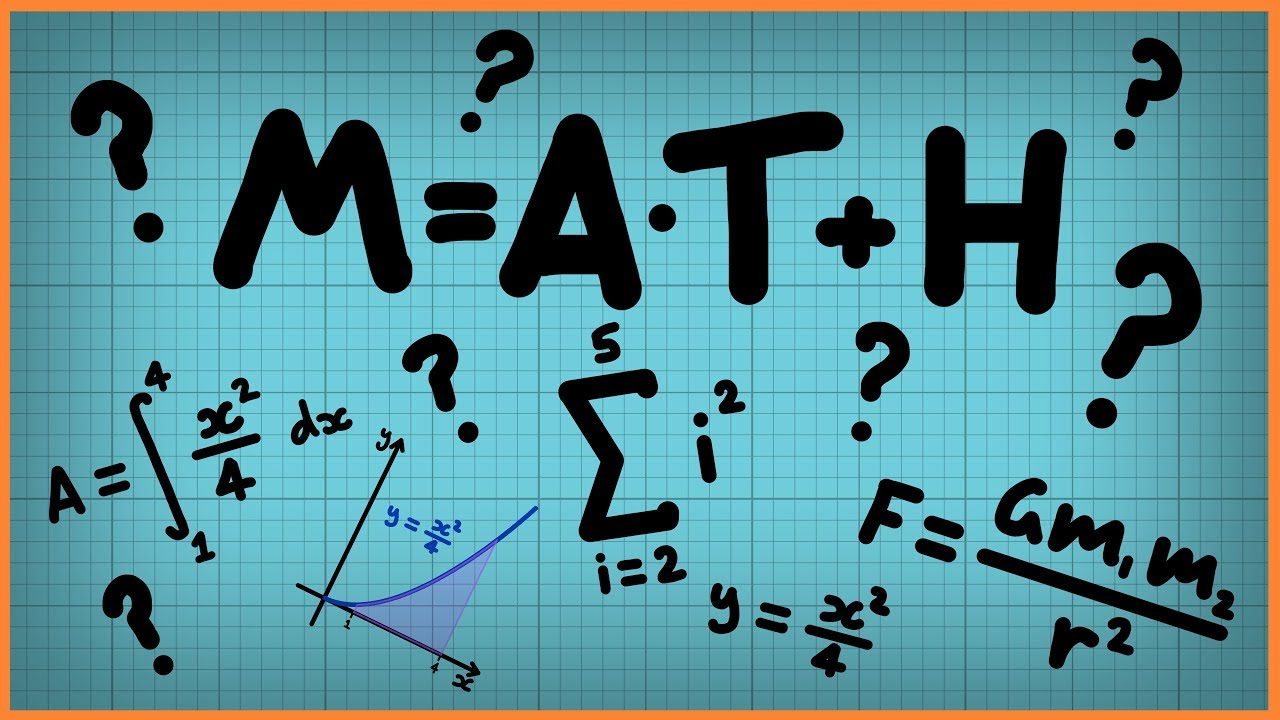Qalaxia Knowlege Bot
0

I found an answer from en.wikipedia.org

Magnetic field - Wikipedia

A magnetic field is a vector field that describes the magnetic influence on moving electric charges, electric currents, :ch1 and magnetized materials. A charge that ...Qalaxia Master Bot
0

Introduction to magnetism (video) | Khan Academy

An introduction to magnetism. ... Magnetic force on a current carrying wire ... When a bar magnet is broken in the middle, what is the effect on its intensity? ... In this time, the Earth's magnetic field automatically aligned the domains, or atoms, of the ... charge) or changing electric fields and create force on moving charges only.Guru Nanak
0

Given that

Number of turns = N

Current = I

Distance between  magnetic field and centre = x

(a) Show that this reduces to the familiar result for field at the centre of the coil.

Magnetic field B = \frac{\mu _0 I R^2 N}{2(x^2 + R^2)^{\frac{3}{2}}}

Magnetic field at centre x = 0

B=\frac{\mu_0IR^2N}{2\left(0^2+\ R^2\right)^{\frac{3}{2}}}

B = \frac{\mu _0 I R^2 N}{2R^3}

B = \frac{\mu _0 I N}{2R}

Hence, B reduces to the familiar result for field at the centre of the coil